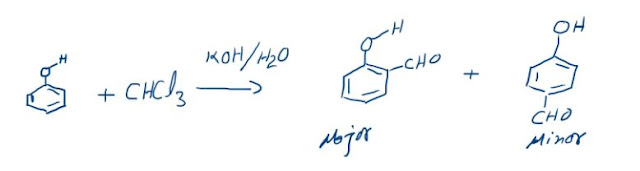### AQA A Level Chemistry Revision Notes

Atomic Structure

• All matter is composed of atoms, which are the smallest parts of an element that can take place in chemical reactions
• Atoms are mostly made up of empty space around a very small, dense nucleus that contains protons and neutrons
• The nucleus has an overall positive charge
• The protons have a positive charge and the neutrons have a neutral charge

• Negatively charged electrons are found in orbitals in the empty space around the nucleus

### Subatomic Particles

• Subatomic particles are the particles an element is made up of and include protonsneutrons and electrons
• These subatomic particles are so small that it is not possible to measure their masses and charges using conventional units (such as grams and coulombs)
• Instead, their masses and charges are compared to each other using ‘relative atomic masses’ and ‘relative atomic charges
• These are not actual charges and masses but they are charges and masses of particles relative to each other
• Protons and neutrons have a very similar mass so each is assigned a relative mass of 1 whereas electrons are 1836 times smaller than a proton and neutron
• Protons are positively charged, electrons negatively charged and neutrons are neutral

### Mass Number & Isotopes

• The mass of an atom is concentrated in the nucleus, because the nucleus contains the heaviest subatomic particles (the neutrons and protons)
• The mass of the electron is negligible

• The nucleus is also positively charged due to the protons
• Electrons orbit the nucleus of the atom, contributing very little to its overall mass, but creating a ‘cloud’ of negative charge
• The electrostatic attraction between the positive nucleus and negatively charged electrons orbiting around it is what holds an atom together
• The number of protons equals the atomic (proton) number
• The number of protons of an unknown element can be calculated by using its mass number and number of neutrons:
• Mass number = number of protons + number of neutrons

Number of protons = mass number - number of neutrons

#### Electrons

• An atom is neutral and therefore has the same number of protons and electrons
• Ions have a different number of electrons to their atomic number depending on their charge
• A positively charged ion has lost electrons and therefore has fewer electrons than protons
• A negatively charged ion has gained electrons and therefore has more electrons than protons

### Isotopes

• Isotopes are atoms of the same element that contain the same number of protons and electrons but a different number of neutrons
• The symbol for an isotope is the chemical symbol (or word) followed by a dash and then the mass number
• E.g. carbon-12 and carbon-14 are isotopes of carbon containing 6 and 8 neutrons respectively
• Isotopes have the same chemical properties but different physical properties

#### Chemical properties

• Isotopes of the same element display the same chemical characteristics
• This is because they have the same number of electrons in their outer shells
• Electrons take part in chemical reactions and therefore determine the chemistry of an atom

#### Physical properties

• The only difference between isotopes is the number of neutrons
• Since these are neutral subatomic particles, they only add mass to the atom
• As a result of this, isotopes have different physical properties such as small differences in their mass and density

• Isotopes are different atoms of the same element that contain the same number of protons and electrons but a different number of neutrons.
• These are atoms of the same elements but with different mass numbers

• Because of this, the mass of an element is given as relative atomic mass (Arby using the average mass of the isotopes
• The relative atomic mass of an element can be calculated by using the relative abundance values
• The relative abundance of an isotope is either given or can be read off the mass spectrum
• Average atomic mass=

### The Reimer-Tiemann reaction: Reaction and its Mechanism

Reimer-Tiemann Reaction Phenols on reaction with chloroform in the presence of sodium hydroxide (or potassium hydroxide) solution give hydro...